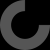MakerBot Print is our newest print-prepration software, which supports native CAD files and STL assemblies,
allows you to interact with all your printers via the Cloud, and many other exciting new features.

Report as inappropriate

I'm putting one of these together and and thought I'd throw this out there for anyone else wondering how to calculate the wind speed.

From what I can tell the diameter of the anemometer is 130 mm and from that you can calculate the speed (not taking into account for drag that of course). Since we know the diameter is 130 mm then we can calculate the circumference to determine the distance traveled in one revolution.

Circumference = Pi x diameter = 3.14159 x 130 = 408.41
Convert that to inches = 408.41 / 25.4 = 16.079 inches

Now we can calculate Wind Speed in mph = RPM x 16.079 x 60 / 5,280
Calculate Knots = mph x 5,280 / 6,080

Example: If you count 100 revolutions per minute (rpm) then our formula is:
100 x 16.079 x 60 / 5,280 = 18.27 mph
or
100 x 16.079 x 60 / 6,080 = 15.87 knots